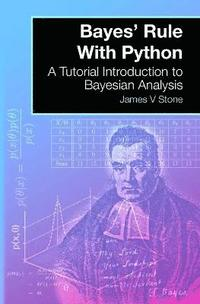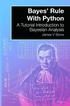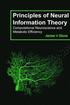Fler böcker inom
Format
Språk
Engelska
Antal sidor
188
Utgivningsdatum
2016-10-15
Förlag
Sebtel Press
Illustrationer
30 Illustrations
Dimensioner
230 x 154 x 10 mm
Vikt
264 g
Antal komponenter
1
Komponenter
423:B&W 6 x 9 in or 229 x 152 mm Perfect Bound on White w/Matte Lam
ISBN
9780993367939

### Du kanske gillar# Bayes' Rule With Python

A Tutorial Introduction to Bayesian Analysis

209
Skickas inom 10-15 vardagar.
Fri frakt inom Sverige för privatpersoner.
Discovered by an 18th century mathematician and preacher, Bayes' rule is a cornerstone of modern probability theory. In this richly illustrated book, a range of accessible examples is used to show how Bayes' rule is actually a natural consequence of common sense reasoning. Bayes' rule is then derived using intuitive graphical representations of probability, and Bayesian analysis is applied to parameter estimation. The tutorial style of writing, combined with a comprehensive glossary, makes this an ideal primer for novices who wish to become familiar with the basic principles of Bayesian analysis. Note that this book includes Python (3.0) code snippets, which reproduce key numerical results and diagrams.

## Passar bra ihop

1.2. +
3.De som köpt den här boken har ofta också köpt Principles of Neural Information Theory av James V Stone (häftad).

## Kundrecensioner

Har du läst boken? Sätt ditt betyg »

## Recensioner i media

"An accessible introduction to Bayesian analysis for those with little mathematical experience."
Journal of the Royal Statistical Society, 2015.

"An excellent book ... highly recommended. "
CHOICE: Academic Reviews Online, February 2014.

"Short, interesting, and very easy to read, Bayes' Rule serves as an excellent primer for students and professionals ... "
Top Ten Math Books On Bayesian Analysis, July 2014.

"An excellent first step for readers with little background in the topic. "
Computing Reviews, June 2014.

"A crackingly clear tutorial for beginners. Exactly the sort of book required for those taking their first steps in Bayesian analysis."
Dr Paul A. Warren.
School of Psychological Sciences, University of Manchester.

"This book is short and eminently readable. It introduces the Bayesian approach to addressing statistical issues without using any advanced mathematics, which should make it accessible to students from a wide range of backgrounds, including biological and social sciences."
Dr Devinder Sivia.
Lecturer in Mathematics, St John's College, Oxford University, and author of Data Analysis: A Bayesian Tutorial.

"For those with a limited mathematical background, Stone's book provides an ideal introduction to the main concepts of Bayesian analysis. "
Dr Peter M Lee.
Department of Mathematics, University of York. Author of Bayesian Statistics: An Introduction.

"Bayesian analysis involves concepts which can be hard for the uninitiated to grasp. Stone's patient pedagogy and gentle examples convey these concepts with uncommon lucidity. "
Dr Charles Fox.
Department of Computer Science, University of Sheffield.

## Innehållsförteckning

Preface

1 An Introduction to Bayes' Rule
1.1 Example 1: Poxy Diseases
1.2 Example 2: Forkandles
1.3 Example 3: Flipping Coins
1.4 Example 4: Light Craters
1.5 Forward and Inverse Probability

2 Bayes' Rule in Pictures
2.1 Random Variables
2.2 The Rules of Probability
2.3 Random Variables and Coin Flips
2.4 Joint Probability and Coin Flips
2.5 Probability As Geometric Area
2.6 Bayes' Rule From Venn Diagrams
2.7 Bayes' Rule and the Medical Test

3 Discrete Parameter Values
3.1 Joint Probability Functions
3.2 Patient Questions
3.3 Deriving Bayes' Rule
3.4 Using Bayes' Rule
3.5 Bayes' Rule and the Joint Distribution

4 Continuous Parameter Values
4.1 A Continuous Likelihood Function
4.2 A Binomial Prior Probability Density Function
4.3 A Posterior Probability Density Function
4.4 A Uniform Prior Probability Density Function
4.5 MAP Estimates Are Not Aected By Constants
4.6 Finding the MAP Estimate Analytically
4.7 Evolution of the Posterior
4.8 Reference Priors
4.9 Loss Functions

5 Gaussian Parameter Estimation
5.1 The Gaussian Distribution
5.2 Estimating the Population Mean
5.3 Error Bars for Gaussian Distributions
5.4 Regression as Parameter Estimation

6 A Bird's-Eye View of Bayes' Rule
6.1 Joint Gaussian Distributions
6.2 A Bird's-Eye View of Joint Distributions
6.3 A Bird's-Eye View of Bayes' Rule
6.4 Slicing Through Joint Distributions
6.5 Statistical Independence

7 Bayesian Wars
7.1 The Nature of Probability
7.2 Subjective Probability
7.3 Bayesian Wars
7.4 A Very Short History of Bayes' Rule

Appendices
A Glossary
B Mathematical Symbols
C The Rules of Probability
D Probability Density Functions
E The Binomial
F The Gaussian
G Least-Squares Estimation
H Reference Priors
I MatLab Code

References

Index